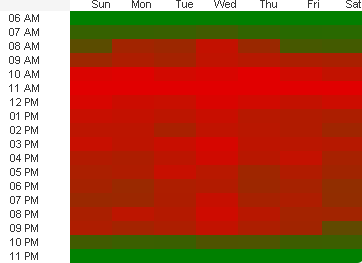# QlikView Layout & Visualizations

Discussion Board for collaboration on QlikView Layout & Visualizations.

Announcements
Coming your way, the Qlik Data Revolution Virtual Summit. October 27-29. REGISTER
cancel
Showing results for
Did you mean:
HighlightedContributor III

## Table as heat map not colored (with colormix) as expected

Hi,

I'm trying to create a heatmap using a pivot table but it isnt showing the colors as expected, and cant find any configuration that can help me.

This is the way I want  the table to be shown. The weekday dim should be the rows and the time dim the columns.Unfortunatly,  the colormix used in the expression background is working vertically instead of horizontally, which is what I need.

As you can see below, if I switch the dims, the colormix does what I need but unfortunatly my client wants to see the table the other way.Has anyone found a way to solve this ?

Best regards,

Diego

1 Solution

Accepted Solutions
HighlightedMVP

Try this

```Aggr(
ColorMix1 ((1+Sign(2*(COUNT( DISTINCT [Transaction #] )-RangeMin (top(total COUNT( DISTINCT [Transaction #] ),1,NoOfRows(total))))/(RangeMax (top(total COUNT( DISTINCT  [Transaction #] ),1,NoOfRows(total)))-RangeMin (top(total COUNT( DISTINCT  [Transaction #] ),1,NoOfRows(total))))-1)*Sqrt(Fabs((2*(COUNT( DISTINCT  [Transaction #] )-RangeMin (top(total COUNT( DISTINCT  [Transaction #] ),1,NoOfRows(total))))/(RangeMax (top(total COUNT( DISTINCT  [Transaction #] ),1,NoOfRows(total)))-RangeMin (top(total COUNT( DISTINCT  [Transaction #] ),1,NoOfRows(total))))-1))))/2, ARGB(255, 0, 128, 0), ARGB(255, 225, 0, 0))
, WeekDay, Time)```
4 Replies
HighlightedMVP
What exactly is your ColorMix expression?
HighlightedContributor III

This is what QV generated:

ColorMix1 ((1+Sign(2*(COUNT( DISTINCT [Transaction #] )-RangeMin (top(total COUNT( DISTINCT [Transaction #] ),1,NoOfRows(total))))/(RangeMax (top(total COUNT( DISTINCT  [Transaction #] ),1,NoOfRows(total)))-RangeMin (top(total COUNT( DISTINCT  [Transaction #] ),1,NoOfRows(total))))-1)*Sqrt(Fabs((2*(COUNT( DISTINCT  [Transaction #] )-RangeMin (top(total COUNT( DISTINCT  [Transaction #] ),1,NoOfRows(total))))/(RangeMax (top(total COUNT( DISTINCT  [Transaction #] ),1,NoOfRows(total)))-RangeMin (top(total COUNT( DISTINCT  [Transaction #] ),1,NoOfRows(total))))-1))))/2, ARGB(255, 0, 128, 0), ARGB(255, 225, 0, 0))

HighlightedMVP

Try this

```Aggr(
ColorMix1 ((1+Sign(2*(COUNT( DISTINCT [Transaction #] )-RangeMin (top(total COUNT( DISTINCT [Transaction #] ),1,NoOfRows(total))))/(RangeMax (top(total COUNT( DISTINCT  [Transaction #] ),1,NoOfRows(total)))-RangeMin (top(total COUNT( DISTINCT  [Transaction #] ),1,NoOfRows(total))))-1)*Sqrt(Fabs((2*(COUNT( DISTINCT  [Transaction #] )-RangeMin (top(total COUNT( DISTINCT  [Transaction #] ),1,NoOfRows(total))))/(RangeMax (top(total COUNT( DISTINCT  [Transaction #] ),1,NoOfRows(total)))-RangeMin (top(total COUNT( DISTINCT  [Transaction #] ),1,NoOfRows(total))))-1))))/2, ARGB(255, 0, 128, 0), ARGB(255, 225, 0, 0))
, WeekDay, Time)```
HighlightedContributor III
It worked, thanks !